# Climate Science Glossary

## Term Lookup

Enter a term in the search box to find its definition.

## Settings

Use the controls in the far right panel to increase or decrease the number of terms automatically displayed (or to completely turn that feature off).

# Settings

All IPCC definitions taken from Climate Change 2007: The Physical Science Basis. Working Group I Contribution to the Fourth Assessment Report of the Intergovernmental Panel on Climate Change, Annex I, Glossary, pp. 941-954. Cambridge University Press.HomeArgumentsSoftwareResources CommentsThe Consensus ProjectTranslationsAbout Support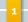Climate's changed before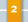It's the sun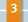It's not bad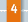There is no consensus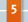It's cooling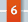Models are unreliable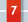Temp record is unreliable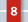Animals and plants can adapt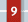It hasn't warmed since 1998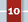Antarctica is gaining ice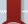View All Arguments...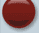## Latest Posts

Archives## Why David Archibald is wrong about solar cycles driving sea levels (Part 1B)

#### Posted on 14 April 2012 by Alex C

After publishing Part 1 (now Part 1A, I suppose), I decided to revisit a concept that crossed my mind early on, but that I had pushed to the back of my mind and the bottom of this series’ to-do list.  However, the issue is important enough that I believe it deserves its own post and special consideration.  Topics that I named and briefly discussed at the end of Part 1A will still be coming in Part 2, but for right now I will focus on the problem of regression.

### Ordinary Least Squares

To briefly cover what some might already know, ordinary least squares regression is a type of linear (y = mx + b) trend-line fitting that minimizes the residuals of your dependent variable from the trend.  The best fit OLS trend can be found by using the below equation: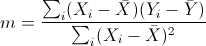and the intercept can be found using the slope and average X/Y values: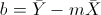For anyone interested in a more detailed explanation on how OLS works, Tamino has a useful and interesting recent post on the subject.  This type of regression is the one that David Archibald and I used, but surprisingly there's actually a wrong way to perform such a simple regression.

### Independent, and Dependent, for a reason

Ordinary least squares is not symmetric.  By non-symmetric, I mean that you cannot flip your axes and obtain the same slope and intercept when you try to find an OLS best-fit with that arrangement.  I said before that OLS minimizes your dependent variable’s residuals, but that’s only an accurate gloss-over of what’s actually happening: you’re minimizing the vertical residuals.  The dependent variable is always plotted on the vertical axis, and de facto you’re minimizing the dependent variable’s residuals.  This is one of the basic assumptions of OLS, that the dependent variable is plotted on the vertical axis.  This is called a regression of “Y on X.”

It’s possible to perform a regression of X on Y, but you have to be aware of what that result is actually telling you.  If you flip your axes so that your dependent is now on the horizontal, and independent on the vertical, you will still obtain a best-fit line, but your slope and intercept (accounting for inverting the slope and intercept on the appropriate axis, due to the inversion of your graph) will not be the same as your original.  Unless all of your data falls on a perfectly straight line, you cannot obtain the same result as before, because what you’re effectively doing now is minimizing your original independent (horizontal) variable’s residuals.  It’s not the same calculation.

To illustrate this, I have developed a dataset of 30 points, randomly generated with a trend imposed on them so that we’ll have a positive slope to work with.  I have fit an OLS trend through the data, and the equation and R2 value for the line are given with it.Figure 1: regression of "Y" on "X," and OLS fit

Figure 2 below is the plot of summed squared vertical residuals for various possible slopes.  This is where I have effectively implemented the brute-force “guess and check” method Tamino alludes to.  The residuals follow a perfect quadratic relationship, as shown by the second-order polynomial fit with a perfect R2 = 1 correlation.  Where the vertex of that parabola is, is where the residuals are at their smallest.  I can give you that value since I have access to the data itself, but we can also calculate the x-value using the equation of the parabola itself: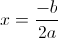The answer is 1.22, which is the slope of the calculated best-fit line Excel gave.Figure 2: minimizing "Y" residuals; residuals defined by deviation from line derived from given slope and above intercept equation; slopes in 0.01 increments

I can also try to find a best-fit slope that minimizes the residuals in the horizontal direction.  The plot of those residuals is below, but the vertical axis is a natural log of the residuals since they get to very high values at very small slopes, and visually this helps to make the minimum more prominent:Figure 3: minimizing "X" residuals; residuals defined by horizontal deviation from best fit line derived from given slope and above intercept equation; slopes in 0.01 increments; natural logarithm applied to residuals for scaling purposes

The minimum is at slope = 1.83.  Is this the slope that we get when we invert the variables and calculate an OLS best-fit?  The figure for that is below.Figure 4: regression of "X" on "Y," and OLS fit

The slopes are not the same – however!  I calculated the x-residuals based on the point-of-view of the variables not being inverted, from the POV that Figure 1 shows.  The slope of the former is “rise over run,” the latter “run over rise” – we have to invert one to compare them, and indeed they do match after such an inversion (1.83 = 1/0.5455).  Inverting the axes has the same effect of minimizing the horizontal residuals.

The inverted slope of the inverted graph (1.83) is not the same as the slope of the non-inverted scatter plot (1.22).  They’re derived from two different calculations.  The non-inverted slope is a regression of Y on X – it tells us how much Y changes per a change in X.  The other, the inverted one, is a regression of X on Y, and tells us how much X changes in response to a change in Y.  The latter assumes that instead of Y being the dependent variable, X is.  By convention, regressions are performed by the dependent on independent, so one needs to choose wisely.

There is one more assumption to OLS regression that deserves mention: that there is no error in the measurements of the independent variable.  The error in measurements is for the dependent variable and describes its deviation about the trend line.  This might not be a valid assumption depending on what data you’re looking at, but for the purposes of the context we’re dealing in, it’s not an issue.

### How this ties in with Archibald’s analysis

David Archibald calculates the “relationship” between solar output and sea level trend by performing a regression of sunspots on sea level trend – sunspots are the “Y” and sea level trend is the “X,” “Y on X.”  He inverts the slope of that best-fit line to give the “quantified” relationship.  This should be setting off some bells and whistles for those that understand the implications of my above explanations.  This regression assumes that it is sea level trend that is the independent variable, and solar output that is dependent; sea level is driving solar output.  The notion is absurd, and while it just so happens that lagging solar output indeed does lead to a better correlation than not (see Part 1A), the regression is likely fallacious from the get-go.

Conventionally, the regression would be performed as sea level trend regressed on solar output, or by having our expected dependent variable (sea level trend) on the vertical and our expected independent variable (sunspots) on the horizontal.  This is fitting from an “X causes Y” perspective, and also because sunspots, in concordance with the second assumption in OLS regression, don’t have any real uncertainty in their values.  We can see sunspots, we count them in real time.  They have virtually no uncertainty; whereas Archibald’s regression would have implied that sea level trend was what had no uncertainty, and solar a normally distributed uncertainty, the conventional regression puts the right uncertainties on the right variables.

I have done that regression, and using the 40-year interval that Archibald chose, we obtain this result:Figure 5: regression of Holgate 2007 sea level trend on sunspots, and OLS fit (data spans 1948-1987 interval)

Using all of the data over the twentieth century:Figure 6: regression of Holgate 2007 sea level trend on sunspots, and OLS fit (data spans 1909-2000 interval)

Compare these results to what we got using the inverted regression:Figure 7: regression of sunspots on Holgate 2007 sea level trend ("inverted" regression), and OLS fit (data spans 1948-1987 interval); original regression performed by David ArchibaldFigure 8: regression of sunspots on Holgate 2007 sea level trend ("inverted" regression), and OLS fit (data spans 1909-2000 interval)

A major foundational point to Archibald’s analysis was the supposed solar “threshold” below which sea level falls.  The 40-year interval gives a "threshold" of 14 sunspots (one third Archibald's result), whereas the "threshold" does not exist when we incorporate all of the data.  We also do not obtain the same trends.  For the 1948-1987 interval, we get a slope of 0.0248mm/yr/sunspot, whereas from the inverted regression we obtain 0.0457mm/yr/sunspot.  For the whole time period, we obtain 0.0118mm/yr/sunspot, and the inverted regression gives 0.0946mm/yr/sunspot.  The former for each is the result we get when we perform the correct regression, while the latter is nonsensical.

It would seem to me that performing a conventional regression, of Y on X, would be the correct thing to do, and would give us the correct slope value.  The inverted regression does not make physical or statistical sense, and I would ask why David Archibald performed such a regression.

(Update, 4-14-12 - minor wording changes for coherence)
(Update, 4-15-12 - equation correction, h/t D_C_S)

1. My guess: Archibald did it both ways, and chose the method that showed the larger "relationship" between sunspots and sea level. Then he got cagey about his language in the hope that nobody would notice. Nice job noticing.
2. I updated the post to make a part early on (about minimizing vertical residuals, or dependent residuals) clearer; it was a change I had made to the structure of the post a few days ago, taking out some sentences, but apparently didn't fully mesh the two parts left over. @kiethpickering #1: I don't think so myself, at the very least for that reason but I don't think so nonetheless. His post tried to demonstrate that sea levels would fall, and whether that happened by -1.whatever mm/year or whether that happened by less (er, more? less in magnitude), it's still a fall.
3. Frankly this was an issue that I didn't fully recognize at first. I had mistakingly regressed the conventional way when I had tried to recreate his own regression, and thought "interesting, why would one be better than the other?" but somewhat dismissed it because I wanted to go on to other topics. It wasn't until I started getting further into my research for these posts that it started to dawn on me that this was a pretty important concept, and that my initial question did have an answer. I think though that if one isn't being too careful, it's a mistake that is rather easy to make.
4. Gosh, a couple edits. Unfortunately my changes made to the post early on weren't reflected in later self-references in the post, so hopefully they're fixed now. Pardon the sloppiness in wording.
5. Alex C, FYI. It's rather easy to show that the OLS Y on X slope (A) and the OLS X on Y slope (B) are mathematically related via the coefficient of determination (R2) according to A*R2 = 1/B. As the R2 approaches 1, the slopes become more and more alike. This is kind of an elementary statistics 101 error. I guess it is one that's easy to make if you are trying a bunch of different things. Still, it's something one should catch.
6. Another way to think about it is that the difference of the slopes is a good indication of the statistical error in the slope's value. If regressing one way gives a different result than regressing the other, there is some uncertainty in the slope value. This is essentially the same as @5, but seems more concrete to me.
7. FWIW, another way to treat this, since there is uncertainty in both X and Y, is to consider (X, Y) to be a Bivariate Normal Distribution, from which the slopes and conditional distributions (of Y|X and X|Y) would pop out naturally without explicitly using regression. The estimated slope of Y|X in the Bivariate Normal is Rsy/sx, the slope of X|Y is Rsx/sy (same as Stephen #5), where s* are the estimates of the univariate standard deviations of X and Y, R the estimated correlation (coefficient of determination). Wikipedia has a good page on the Bivariate Normal.
8. Sorry, have been away from my computer for a while: @Stephen Baines #5: Perhaps you mean A/R^2 = 1/B (AB = R^2)? It is that equation that seems to work, when I multiply the CoD by A I don't get 1/B (e.g. 0.12501*10.575 ≠ 1/.0118). As to it being an error, were you referring to an error I made, or Archibald's error? Thanks for the FYI either way though, I assumed they were relatable but didn't know the simplified form to do so. @shoyemore: It's not clear that there's any uncertainty in sunspot counts, certainly not normally distributed uncertainty. As I said in the post, we can see sunspots and count them in realtime.
9. @_rand_ It's true that the difference between the (slope of Y on X)and (1/(slope of X on Y)) is correlated to the error in the slope. Just to be clear, though, that does not mean that either formulation is equally correct given. Archibald just gets it wrong. He first regresses Y on X and then uses that equation to predict Y from X. As long as there is significant error in the relationship OLS will never give you the right answer if you do that. shoyemore It would make sense to calculate the bivariate normal slope (its really only one slope that can be inverted depending on which variable is on the X and Y axis) if 1)you really though of this relationship as a correlation, where one variable really does not control the other and 2) you wanted to characterize an underlying central tendency between two related but otherwise independent variables. However, you would not then use that slope to predict Y from X. That is the specific purpose OLS was designed for - making the most precise predictions of Y given a value for X. It does so by finding a line that minimizes residuals for Y. As long as you don't predict outside the range of the original data, OLS will do fine. The bivariate normal slope, on the other hand, does not minimize the residuals for Y, and so is not the best predictor line. That's not really it's purpose though. In any case, what Archibald did was even worse. He ran the OLS regression of X on Y and then use to predict Y as if he has run it Y on X. That will always fail to produce an accurate prediction. You are predicting Y from a relationship that minimize errors in predicting X. It makes no sense.
10. Alex C...A is the Y on X slope, so it should be lower that 1/(X on Y slope), no? No matter...I think we're just getting our A's and B's and our Y's and X's mixed up. In any case, the two are related via R2. And the mistake is clearly Archibald's...sorry about the confusion. And the typos!
11. Alex C, the assumption that sunspot numbers do not have any error is incorrect. There are at least six difficulties with observing sunspots that can lead to error. The first, and most obvious is that sunspots on the far side of the Sun cannot be observed. The period of solar rotation is 23 days in mid latitude regions, while sunspots can las from "just a few hours" to months, with the longest lasting "about six months". Therefore some sunspots can appear and then cease to exist entirely on the opposite side of the Sun from the Earth, and hence be unobserved. The second is that sunspots are very varied in size, and in the brightness of their background making counting of a sunspot a matter of judgement, which of course may vary among observers. To illustrate this point, consider the sunspot group below:(Image from wikipedia) Do you count only the very dark areas as sunspot, or the dark grey regions? If only the very dark areas (which is my supposition), or the larger dark grey areas? If the darker grey area, there will be significant issues of observability based on telescope quality. If the the very dark areas, do you count the the large extended region on the upper right of the group as one spot, because it is continuous , or as two because of the obvious necking between the left and right regions of the group? On a similar basis, do you count the long thin vertical dark region on the upper left as 1, 2 or 3 sunspots on a similar basis? Further, what of the very small dark spots on the within the darker background? Are they distinct sunspots, or just part of the general variation of darkness within that background? Third, obviously the observational questions above are made more difficult for sunspot groups observed near the limb of the Sun, and also when observational conditions are poor due to weather (as sunspot observations are made by ground based telescopes). Fourth, historically sunspot observations have not been complete. In particular, prior to 1849 up to twenty days may be missing from any given months observations. To compensate for these issues, sunspots are measured by using the Wolf Number, which is K(10g+s), where g is the number of groups, and s is the number of sunspots observed. g is multiplied by 10 because that is the average number of spots per group, but that is an obvious additional source of potential error when we take the Wolf number as a direct index of solar activity. K is the "personal reduction coefficient":
"A personal reduction coefficient K is calculated for each station. It has values typically in the range 0.4 and 1.7 and leads to a normalized sunspot number K(10G+S). In other words, the K factor rescales the raw sunspot counts of each observer to the ones of Rudolph Wolf, the astronomer who introduced the above Wolf formula, thus simulating the same eyes, same telescope and same conditions."
(Source) Although scaling by K is necessary for a consistent index, again it is a potential source of error. Despite the scaling for groups and the use of a personal reduction coefficient introduces potential errors, by compensating for the other factors above, I assume they result in a smaller overall error in the index.
12. @Tom Curtis #11: (I assume the compensation with the Wolf Number, and K, are the fifth and sixth potential sources of error?) Very informative, I was acting off a much more simplified understanding of sunspot collection and thus stand corrected. OLS assumes zero uncertainty in the independent variable, so perhaps a more appropriate form of regression would be a total least squares regression? From what little I understand of the issue, performing a Deming regression would be fairly simple. If I am not mistaken (and I might be), the result would be some middle ground between the Y|X and X|Y regressions, depending on the ratio of the variances in the variables.
13. Alex C @12, yes, the compensation (10 times multiplier on groups) and K are the fifth and sixth potential sources of error. I finally manage to find an error stated on the sunspot number, +/-5% (see page 2 of the bulletin) I assume that if the error on the independent variable is small compared to that on the dependent variable, OLS will still provide a good estimate. On the assumption that that is not true, does Total Least Squares Regression assume approximately equal percentage error for both variables, or is it independent of the error? Further, how would using TLSR effect your analysis above? And finally, what form of regression did Archibald actually use?
14. @Tom Curtis #13: Deming regression from what I can tell can be performed using knowledge of the ratio of variances between the datasets, which I don't know and which I am unfamiliar with how to coax out of cyclical - yet uneven - data as sunspots. There is also an issue with the sea level trend data and how it was processed, the 10-year moving derivative amplifies a frequency range in which the solar cycle frequency (~0.9) lies, so I don't know if I could apply the same sort of methodology to sea level as I could to solar. Whatever that methodology is anyway. As to how it would change it, if we do assume for a minute equal variance (due to how we don't know the variances), then the residuals should be minimized perpendicular to the line, in which case the result is hardly different at all from the conventional method. I think my analysis above holds, but I'd be happy to hear from someone with more experience on this.
15. Alex C, I wouldn't overthink this. OLS will provide the best linear model if the aim is to simply predict Y from X, even in the presence of error in X. Where error in X rears its ugly head is when you want to compare the slopes in the OLS model to some theoretical expectation. Such theoretical expectations assume that you know the x variable precisely. The OLS slope will therefore be biased low compared to that theoretical slope. If we know the error variance in X (the variance associated with measuerement error), the bias in the slope can be calculated from the ratio between the error variance in X and total variance in X.
16. Alex C & Stephen Baines Thanks for the clarifications. No issue here.
17. Timely article considering I've been dealing with using regression for model evaluation of observed and predicted variables in a paper I'm working on publishing. Albeit regression doesn't provide a singular statistic to indicate performance while validating a model (check out concordance correlation coefficient instead) it's funny just how often people mix up the axes, but actually end up being correct in their "mistake". It all depends on what you're working with though. It's a topic most people just skim over. Anyone with journal access might want to give this paper a read if this topic is interesting them: Piñeiro et al. 2008. How to evaluate models: Observed vs. predicted or predicted vs. observed? Ecological Modelling: Volume 216, Issues 3–4, 10 September 2008, Pages 316–322. The issue of "predicted vs. observed" doesn't pertain to what Archibald was doing from what I can see, but the article demonstrates how a lot of the topics work that are being hit on here.
18. The points (x,y) = (1,2) & (3,6) are on the line y = 2 x. I put these points into the formula that you gave for m, which resulted in a value for m of 1/2 instead of 2. You must have your X's & Y's switched in the formula if you are looking for an equation of the form y = m x + b.
19. @D_C_S #18: Got to love it when errors are pointed out after publication, rather than before :-) You are correct, the slope is found by dividing by the variance in the independent variable, hence ∑(x_i - x_bar)^2, not y. Horribly sloppy on my part, having a hard time believing that I did an entire post based around the same concept and mistake. I will make the change. In case anyone would like a good source I found informative and fairly easy to read, this helps explain the concepts I've covered, and gives the equations: http://www.edwardtufte.com/tufte/dapp/DAPP3a.pdf